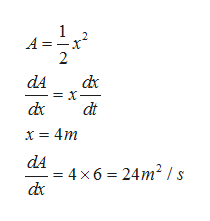# The legs of an isosceles right triangle increase in length at a rate of 6 m divided by s.a. At what rate is the area of the triangle changing when the legs are 4 m​ long?b. At what rate is the area of the triangle changing when the hypotenuse is 6 m​ long?c. At what rate is the length of the hypotenuse​ changing?

Question
8 views

The legs of an isosceles right triangle increase in length at a rate of 6 m divided by s.
a. At what rate is the area of the triangle changing when the legs are 4 m​ long?
b. At what rate is the area of the triangle changing when the hypotenuse is 6 m​ long?
c. At what rate is the length of the hypotenuse​ changing?

check_circle

star
star
star
star
star
1 Rating
Step 1

Let x  be the length of leg and h be the length of hypotenuse of an isosceles right triangle. Then we have

Step 2

Now, We know that Area of right angled triangle is 1/2 of product of length of legs , so we havehelp_outlineImage Transcriptionclose1 A = dxc = x- dc dA dt x = 4m dA =4x6 24m2/s dx fullscreen
Step 3

If length of hypotenuse is 6m, then we c...

### Want to see the full answer?

See Solution

#### Want to see this answer and more?

Solutions are written by subject experts who are available 24/7. Questions are typically answered within 1 hour.*

See Solution
*Response times may vary by subject and question.
Tagged in

### Derivative